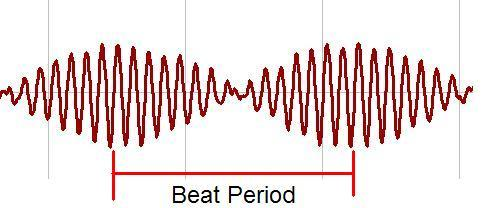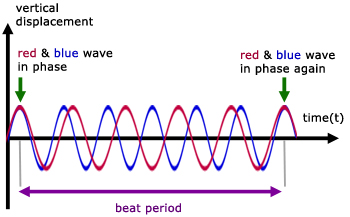Click to Chat

1800-1023-196

+91-120-4616500

CART 0

• 0

MY CART (5)

Use Coupon: CART20 and get 20% off on all online Study Material

ITEM
DETAILS
MRP
DISCOUNT
FINAL PRICE
Total Price: Rs.

There are no items in this cart.
Continue Shopping• Complete Physics Course - Class 11
• OFFERED PRICE: Rs. 2,968
• View Details

```BeatsWhen two waves of nearly equal frequencies travelling in a medium along the same direction superimpose upon each other, beats are produced. The amptitude of the resultant sound at a point rises and falls regularly.

The intensity of the resultant sound at a point rises and falls regularly with time. When the intensity rises to maximum we call it as waxing of sound, when it falls to minimum we call it as waning of sound.

The phenomenon of waxing and waning of sound due to interference of two sound waves of nearly equal frequencies are called beats. The number of beats produced per second is called beat frequency, which is equal to the difference in frequencies of two waves.

Analytical methodLet us consider two waves of slightly different frequencies n1 and n2 (n1 ~ n2 < 10) having equal amplitude travelling in a medium in the same direction.

At time t = 0, both waves travel in same phase. The equations of the two waves are

y1 = a sin ω1t

y1 = a sin (2πn1)t         …... (1)

y2 = a sin ω2t

= a sin (2πn2)t              …... (2)

When the two waves superimpose, the resultant displacement is given by

y =  y1 + y2

y = a sin (2πn1)t + a sin (2πn2)t        …... (3)

Therefore

y = 2a sin 2π(n1+ n2/2)t cos 2π(n1– n2?/2)t            …... (4)

Substitute A = 2a cos 2π(n1– n2?/2)t   and n = n1 + n2/2 in equation (4)

y = A sin 2πnt

This represents a simple harmonic wave of frequency n = n1 + n2/2 and amplitude A which changes with time.

(i) The resultant amplitude is maximum (i.e) ± 2a, if

cos 2π [n1-n2/2] t = ±1

So, 2π [n1-n2/2] t = ±mπ

(Here, m = 0,1,2....) or (n1 – n2)t = m

The first maximum is obtained at t1 = 0

The second maximum is obtained at,

t2 = 1/n1 – n2

The third maximum at t3 = 2/n1 – n2 and so on.

The time interval between two successive maxima is,

t2 – t1 =  t3 – t2 = 1/n1 – n2

Hence the number of beats produced per second is equal to the reciprocal of the time interval between two successive maxima.(ii) The resultant amplitude is minimum (i.e) equal to zero, if

cos 2π [n1-n2/2] t = 0

(i.e) 2π [n1-n2/2] t = π/2 + mπ = (2m+1)π/2

Or, [n1-n2/2] t = (2m+1)/2

Where m = 0, 1, 2...

The first minimum is obtained at,

t1' = 1/2(n1 – n2)

The second minimum is obtained at,

t2' = 3/2(n1 – n2)

The third minimum is obtained at,

t3' = 5/2(n1 – n2) and so on

Time interval between two successive mimima is

t2' –  t1' = t3' – t2' = 1/n1 – n2

Hence, the number of beats produced per second is equal to the reciprocal of time interval between two successive minima.

Refer this video to know more about on beats

Uses of beats

(i) The phenomenon of beats is useful in tuning two vibrating bodies in unison. For example, a sonometer wire can be tuned in unison with a tuning fork by observing the beats. When an excited tuning fork is kept on the sonometer and if the sonometer wire is also excited, beats are heard, when the frequencies are nearly equal. If the length of the wire is adjusted carefully so that the number of beats gradually decreases to zero, then the two are said to be in unison. Most of the musical instruments are made to be in unison based on this method.

(ii) The frequency of a tuning fork can be found using beats. A standard tuning fork of frequency N is excited along with the experimental fork. If the number of beats per second is n, then the frequency of experimental tuning fork is N+n. The experimental tuning fork is then loaded with a little bees’ wax, thereby decreasing its frequency. Now the observations are repeated. If the number of beats increases, then the frequency of the experimental tuning fork is N-n, and if the number of beats decreases its frequency is N + n.The number of beats per second equals the difference in frequency between the two interfering waves.

The beat frequency is equal to the absolute value of the difference in frequency of the two waves.

Sound is a pressure wave that consists of compressions and rarefactions.

The interference of sound waves causes the particles of the medium to behave in a manner that reflects the net effect of the two individual waves upon the particles.

The beat frequency refers to the rate at which the volume is heard to be oscillating from high to low volume.

The beat frequency is always equal to the difference in frequency of the two notes that interfere to produce the beats.

When two waves of similar frequencies interfere, the result is a beat frequency.

A beat frequency is a pulsing sound that goes up and down in loudness.

As the two waves go in and out of phase, the varying constructive and destructive interference makes the wave grow and shrink in amplitude. For sound waves this produces a beating sound.

Second order beats occur between the two notes of a mistuned octave, and binaural beats involve beating between tones presented separately to the two ears, so that they do not mix physically.

Problem (JEE Main):

Two tuning forks A and B when sounded together produce 4 beats. If A is in unison with the 0.96 m length of a sonometer wire under a tension, B is in unison with 0.97 m length of the same wire under same tension. Calculate the frequencies of the forks.

Data : l1 = 0.96 m; l2 = 0.97 m; n1 = ?; n2 = ? l1 < l2 ∴ n1 > n2

Solution:

Let n1 = n  and  n2 = n - 4

According to 1st law of transverse vibrations

n1 l1 = n2 l2

n × 0.96 = (n-4) × 0.97 n(0.97 - 0.96) = 3.88

n = 3.88/0.01= 388 Hz

n2 = 388 - 4 = 384 Hz

The frequency of the fork A is n1 = 388 Hz,

The frequency of the fork B is n2 = 384 Hz.Question 1

The main factor which effects the speed of a sound wave is the ____.

(a) amplitude of the sound wave               (b) intensity of the sound wave

(c)  properties of the medium                 (d)  loudness of the sound wave

Question 2

The ____ is defined as the number of cycles of a periodic wave occurring per unit time.

(a) wavelength       (b) period

(c) amplitude         (d) frequency

Question 3

The number of beats produced per second is equal to

(a) the sum of the frequencies of two tuning forks

(b) the difference of the frequencies of two tuning forks

(c) the ratio of the frequencies of two tuning forks

(d) the frequency of either of the two tuning forks

Question 4

Beats are the results of

(a) diffraction of sound waves          (b) constructive and destructive interference

(c) polarization                              (d) destructive interference

Question 5

When the source of sound moves away form a stationary listener then _________ occurs.

(a) an apparent decrease in frequency

(b) an apparent increase in frequency

(c) an apparent decrease in wavelength

(d) no apparent change in frequencyQ.1
Q.2
Q.3
Q.4
Q.5

c

d

b

b

a

Related Resources:-

You might like to refer mechanical wave motion.

For getting an idea of the type of questions asked, refer the  Previous Year Question Papers.

To read more, Buy study material of Waves comprising study notes, revision notes, video lectures, previous year solved questions etc. Also browse for more study materials on Physics here.

```### Course Features

• 101 Video Lectures
• Revision Notes
• Previous Year Papers
• Mind Map
• Study Planner
• NCERT Solutions
• Discussion Forum
• Test paper with Video Solution